Next: 2.4.3 Common kernel functions Up: 2.4 Kernel PCA Previous: 2.4.1 Feature extraction

## 2.4.2 Centering in feature space

So far, we have assumed that {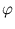(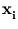)} has zero mean, which is usually not fulfilled. Therefore, the formalism needs to be adjusted (Schölkopf et al., 1998b). The following set of points will be centered: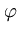(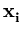) =() -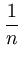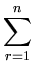(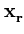) . (2.31)

The above analysis holds if the covariance matrix is computed from(). Thus, the kernel matrix Kij =()T(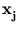) needs to be replaced by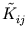=()T(). Using (2.31),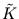can be written as,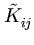=()T(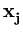) -()T() -()T() +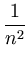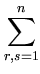()T(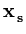) = Kij -Kir -Krj +Krs . (2.32)

Therefore, we can evaluate the kernel matrix for the centered data using the known matrix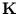. For the remainder of this thesis, I denote with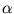the eigenvectors of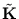instead of, and they are normalized according to (2.29) using the eigenvalues of. The principal components are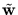=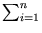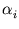().Next: 2.4.3 Common kernel functions Up: 2.4 Kernel PCA Previous: 2.4.1 Feature extraction
Heiko Hoffmann
2005-03-22